• ## Pandas DataFrame的基本属性详解

万次阅读 多人点赞 2019-06-24 19:13:33
Pandas DataFrame的一些基本属性 基本功能列表 import pandas as pd 导入库 df = pd.DataFrame(data=None, index=None, columns=None, dtype=None, copy=False) 创建一个DataFrame df.index df.columns df.axes df.T...
注：以下代码均在Jupyter中运行的。
基本功能列表
import pandas as pd          导入库
df = pd.DataFrame(data=None, index=None, columns=None, dtype=None, copy=False)
创建一个DataFrame

代码
功能

DataFrame()
创建一个DataFrame对象

df.values
返回ndarray类型的对象

df.iloc[ 行序,列序 ]
按序值返回元素

df.loc[ 行索引,列索引 ]
按索引返回元素

df.index
获取行索引

df.columns
获取列索引

df.axes
获取行及列索引

df.T
行与列对调

df. info()
打印DataFrame对象的信息

df.head(i)
显示前 i 行数据

df.tail(i)
显示后 i 行数据

df.describe()
查看数据按列的统计信息

创建一个DataFrame
DataFrame()函数的参数index的值相当于行索引，若不手动赋值，将默认从0开始分配。columns的值相当于列索引，若不手动赋值，也将默认从0开始分配。
data = {
'性别':['男','女','女','男','男'],
'姓名':['小明','小红','小芳','大黑','张三'],
'年龄':[20,21,25,24,29]}
df = pd.DataFrame(data,index=['one','two','three','four','five'],
columns=['姓名','性别','年龄','职业'])
df

运行结果：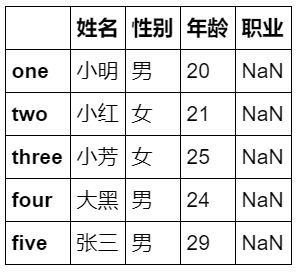df.values 返回ndarray类型的对象
ndarray类型即numpy的 N 维数组对象,通常将DataFrame类型的数据转换为ndarray类型的比较方便操作。如对DataFrame类型进行切片操作需要df.iloc[ : , 1:3]这种形式，对数组类型直接X[ : , 1:3]即可。
X = df.values
print(type(X)) #显示数据类型
X

运行结果：
<class 'numpy.ndarray'>
[['小明' '男' 20 nan]
['小红' '女' 21 nan]
['小芳' '女' 25 nan]
['大黑' '男' 24 nan]
['张三' '男' 29 nan]]

df.iloc[ 行序,列序 ] 按序值返回元素
df.iloc[1,1]

运行结果：
Index(['one', 'two', 'three', 'four', 'five'], dtype='object')

df.loc[ 行索引,列索引 ] 按索引返回元素
df.loc['one','性别']

运行结果：
男

df.index 获取行索引
df.index

运行结果：
Index(['one', 'two', 'three', 'four', 'five'], dtype='object')

df.columns 获取列索引
df.columns

运行结果：
Index(['姓名', '性别', '年龄', '职业'], dtype='object')

df.axes 获取行及列索引
df.axes

运行结果：
[Index(['one', 'two', 'three', 'four', 'five'], dtype='object'),
Index(['姓名', '性别', '年龄', '职业'], dtype='object')]

df.T index 与 columns 对调
df.T

运行结果：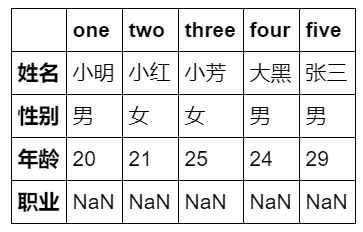df.info() 打印DataFrame对象的信息
df.info()

运行结果：
<class 'pandas.core.frame.DataFrame'>
Index: 5 entries, one to five
Data columns (total 4 columns):
姓名    5 non-null object
性别    5 non-null object
年龄    5 non-null int64
职业    0 non-null object
dtypes: int64(1), object(3)
memory usage: 200.0+ bytes


df.head(i) 显示前 i 行数据
df.head(2)

运行结果：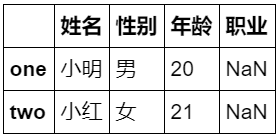若想要显示前几列数据，可用df.T.head(i)
df.tail(i) 显示后 i 行数据
df.tail(2)

运行结果：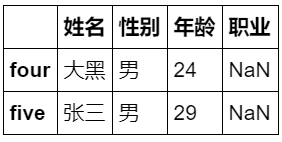df.describe() 查看数据按列的统计信息
可显示数据的数量、缺失值、最小最大数、平均值、分位数等信息
             年龄
count   5.000000
mean   23.800000
std     3.563706
min    20.000000
25%    21.000000
50%    24.000000
75%    25.000000
max    29.000000



展开全文Python Pandas
• 首先新建一个Dataframe import pandas as pd df = pd.DataFrame({'a':[1,2,3],'b':[1,2,3]}) 如下： a b 0 1 1 1 2 2 2 3 3 1、修改列名a，b为A、B。 df.columns = ['A','B'] 2、只修改列名a为A
首先新建一个Dataframe

import pandas as pd

df = pd.DataFrame({'a':[1,2,3],'b':[1,2,3]})

如下：
a  b
0  1  1
1  2  2
2  3  3

1、修改列名a，b为A、B。

df.columns = ['A','B']

2、只修改列名a为A

df.rename(columns={'a':'A'})

如你想了解更多关于python和spark以及机器学习的知识，可以关注下面的公众号我们一起沟通交流。展开全文python pandas
• 不得不说DataFrame现在很火，已经有很多库都是基于DataFrame写的，而且它用起来也很方便，读excel只需要一行代码，起使用xlrd的日子，至今还脑壳疼，所以对于一个用python做数据处理的人来说，pandas是必须要了解的...
不得不说DataFrame现在很火，已经有很多库都是基于DataFrame写的，而且它用起来也很方便，读excel只需要一行代码，想起使用xlrd的日子，至今还脑壳疼，所以对于一个用python做数据处理的人来说，pandas是必须要了解的。对于一个数据处理工具来说，读写是最基本的，下面是我最近整理的关于pandas一些基本本操作，主要包括以下内容：
如何创建DataFrame
如何读取DataFrame的值，读一行/列、读多行/列
如何对DataFrame赋值
如何对DataFrame插入一（多）行/列
如何删除DataFrame的一（多）行/列
开始前先引入两个库
import pandas as pd
import numpy as np

1 创建DataFrame
1.1 利用字典创建
data={"one":np.random.randn(4),"two":np.linspace(1,4,4),"three":['zhangsan','李四',999,0.1]}
df=pd.DataFrame(data,index=[1,2,3,4])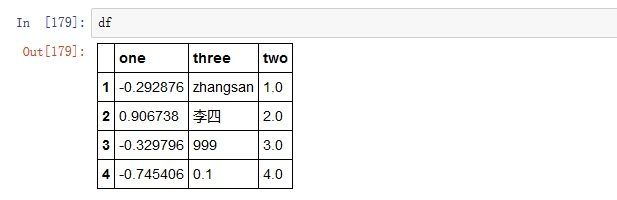如果创建df时不指定索引，默认索引将是从0开时，步长为1的数组。
df的行、列可以是不同的数据类型，同行也可以有多种数据类型。
df创建完成后可以重新设置索引，通常用到3个函数：set_index、reset_index、reindex。
set _index用于将df中的一行或多行设置为索引。df.set_index(['one'],drop=False) ordf.set_index('one)df.set_index(['one','two'])
参数drop默认为True，意为将该列设置为索引后从数据中删除，如果设为False，将继续在数据中保留该行。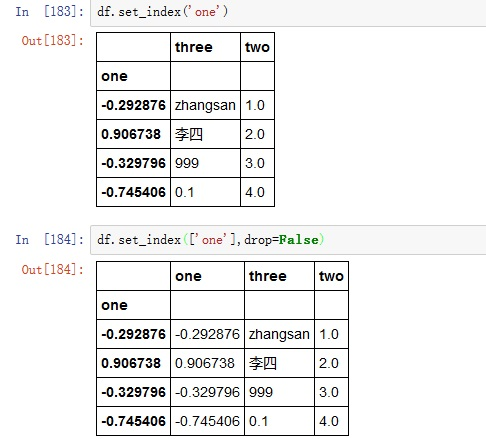如果要设置的索引不在数据中，可以通过df.index=['a','b','c','d']
reset_index用于将索引还原成默认值，即从0开始步长为1的数组。df.reset_index(drop=True)
参数drop默认值为False，意为将原来的索引做为数据列保留，如果设为True，原来的索引会直接删除。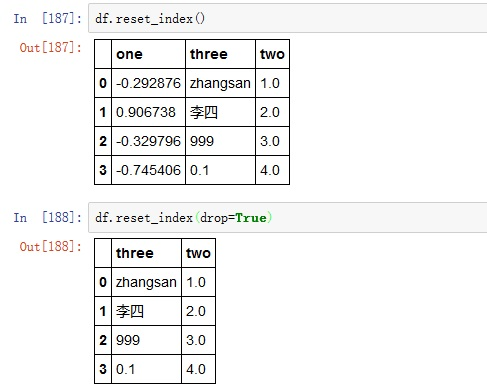reindex比较复杂，也不常用到，这里是基础篇，不做大量说明，感兴趣的朋友可以看官方文档
1.2 利用数组创建
data=np.random.randn(6,4)#创建一个6行4列的数组
df=pd.DataFrame(data,columns=list('ABCD'),index=[1,2,'a','b','2006-10-1','第六行'])

1.3 创建一个空DataFrame
pd.DataFrame(columns=('id','name','grade','class'))

为了便于理解，以下面DataFrame为例，对其读写操作展开说明：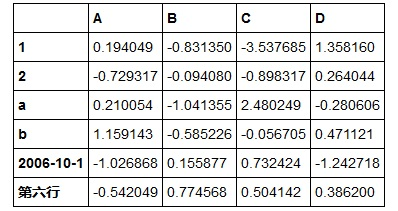2 读DataFrame
2.1按列读取
方法1：df.列名
该方法每次只能读取一列。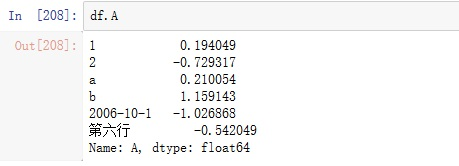方法2：df['列名']or df[['列名']]、df[['列名1','列名2','列名n']]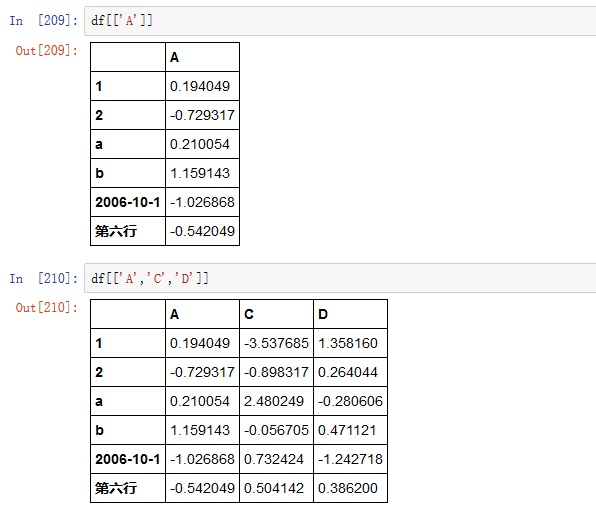ps：df['A']和 df[['A']]都能读取第一列数据，但它们的返回结果是不同的，这里的不同指的是数据结构的不同，感兴趣的朋友可以用type(df['A']),type(df[['A']])查看。方法3 .iloc[:,colNo] or .iloc[:,colNo1:colNo2]
按列号读取，有时候我们可能更希望通过列号（1，2，3…）读取数据而不是列名，又或着我们要读取多行的时候一个一个输入列名是很麻烦的，我们希望有最简单的代码读取我们最想要的内容，.iloc方法可以让我们通过列号索引数据，具体如下：df.iloc[:1]读取第一列df.iloc[:,1:3]读取第1列到第3列df.iloc[:,2:]读取第2列之后的数据df.iloc[:,:3]读取前3列数据ps:这其实是按单元格读取数据的特殊写法，如果有疑问请看 2.3 按单元格读取数据。
2.2 按行读取
方法1：.loc['行标签']or.loc[['行标签']]、.loc[['行标签1','行标签2','行标签n']].loc根据行标签索引数据，这里的行标签可以理解为索引（没有深入研究，但是在这里，行标签=索引）,比如我们要分别读取第1行和第3行就是df[]、df[['a']]，如果该df的索引变为['a', 'b', 'c', 'd', 'e', 'f']，分别读取第1行和第3行的操作将变成df[['a']],df[['c']]，也就是说.loc后面的'行标签'必须在索引中。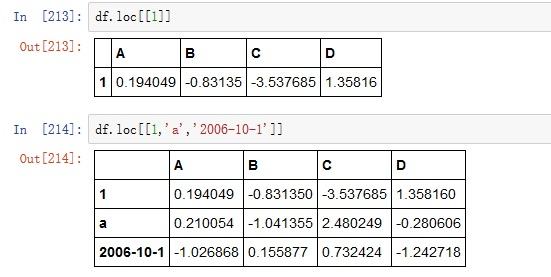ps：df.loc[]和df.loc返回结果的值相同，但数据结构有差异方法2：.iloc['行号']or.iloc[['行号']]、.iloc[['行号1','行号2','行号n']].iloc根据行号索引数据，行号是固定不变的，不受索引变化的影响，如果df的索引是默认值，则.loc和.iloc的用法没有区别，因为此时行号和行标签相同。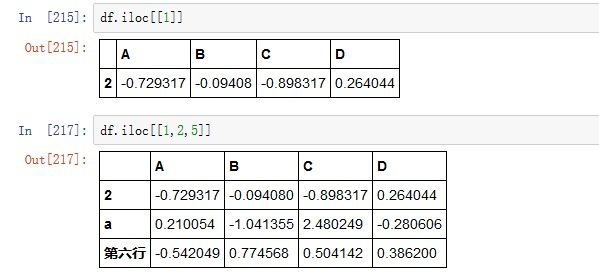可以看到df.loc和df.iloc读到的内容是不一样的，df.loc读取的是索引号为1的那一行，df.iloc读取的是第1行。
此外，.iloc可以通过切片的方式读取数据，所谓切片就是给出要读数据的首尾位置，然后读取首尾中间这“一片”数据（个人理解，可能理解的不对或比较片面，对此有疑惑的朋友请自行查阅相关资料）比如我们要读取第1行到第4行的数据，利用切片的方法就是df.iloc[1:5]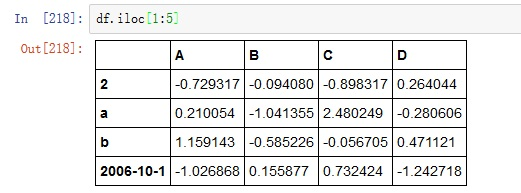如果从第0行开始读，则首位置可以省略，如果从某一行读到末尾，则尾位置可以省略。df.iloc[:5]，读取第0行到第4行的数据；df.iloc[8:]，读取第8行后所有数据，包括第8行；df.iloc[3,6]，读取第3行到第6行的数据，包括第3行但不包括第6行。方法3：.ix
根据其他网友的说法，.ix是.loc和.iloc的综合版，既可以通过行标签检索也可以根据行号检索。通过实验发现这个说法并不完成正确。以上面的df为例：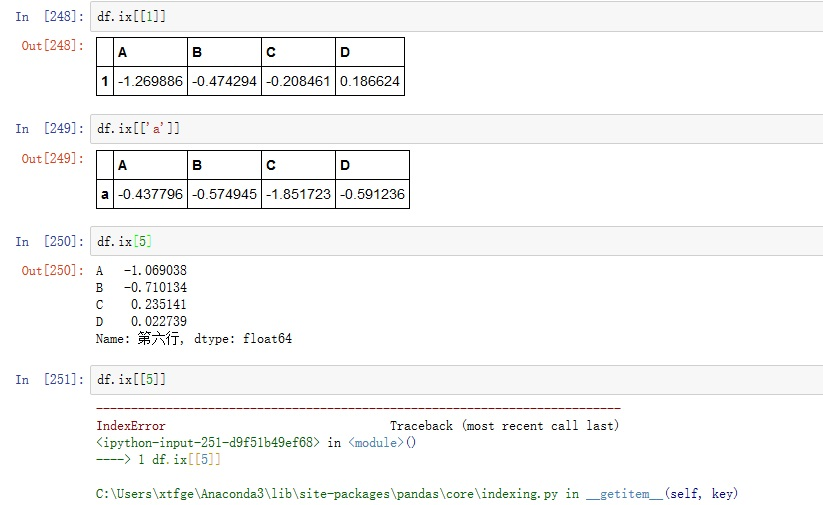因为df.ix[]可以认为是要读第1行，也可以认为要读索引为1的那一行，此时就产生了冲突，可以看到此时pandas认为df.ix[]读的是索引为1的那一行，如果用索引外的标签检索就会报错，而df.ix被认为是第1行，此时df.ix和df.ix[]读到的不是同一行数据。
如果我们将df的索引改为['a', 'b', 'c', 'd', 'e', 'f']再进行上面的操作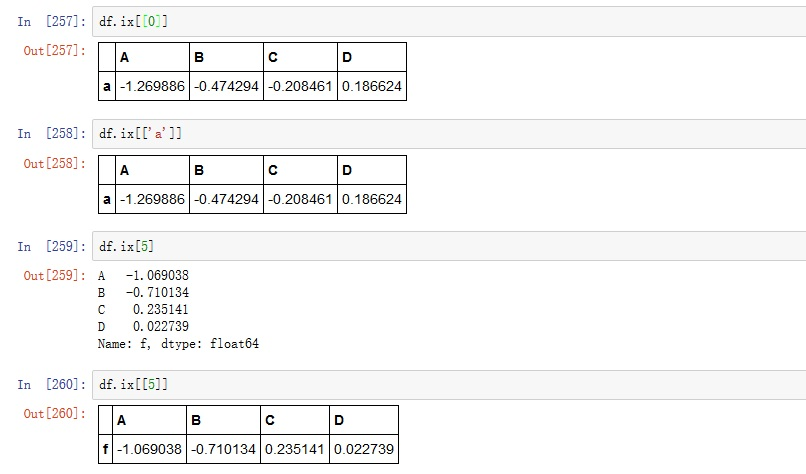此时df.ix和df.ix[]读到的是同一行数据。
通过以上实验，loc，iloc，ix的区别如下：loc通过索引标签读取数据；iloc通过行号读取数据；ix既可以通过行号读取数据，也可以通过索引标签读取数据，但是当索引为数字且不从0开始时，有两种情况：
（1）每次读一行：通过索引标签读取和通过行号读取有不同的写法.ix[['列标签']],.ix[行号]。
（2）读取多行：此时只能通过索引标签，不能利用行号。方法4：at、iatat、iat这里就不做介绍了，因为上面的方法完全够用了，感兴趣的话可以看官方文档。.loc,.iloc完全可以满足DataFrame的读取操作，所以ix,at,iat并不推荐使用。
2.3 按单元格读取
方法1：df[col][row]
读取一个单元格的数据时推荐使用，也可以写成df.col[row]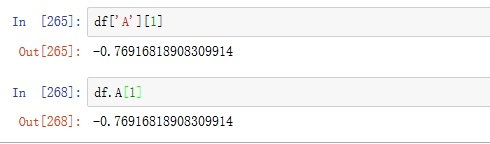方法2：.loc
(1)读取一个单元格：df.loc[row][col]或df.loc[row,col]
(2)读取一行多列：
df.loc[row][[col1,col2]]
df.loc[1,[col1,col2]]
df.loc[row][firstCol:endCol]
df.loc[row,firstCol:endCol]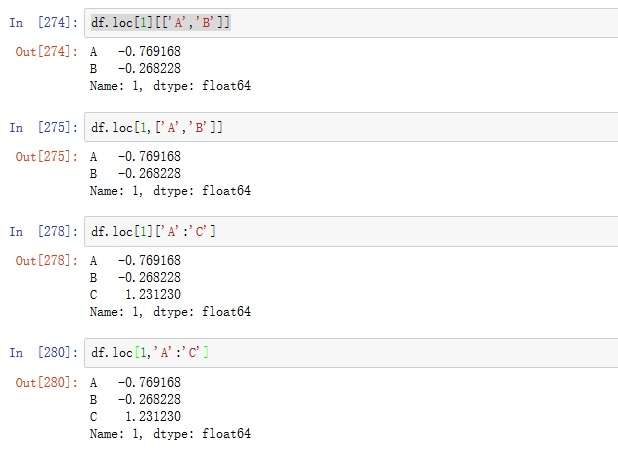(3)读取多行一列：
df.loc[[row1,row2]][col]
df.loc[[row1,row2]].col
df.loc[[row1,row2],col]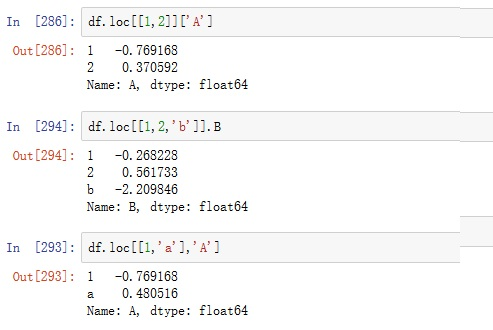ps :在这里行号不能用切片。
(4)读取多行多列
df.loc[[row1,row2],[col1,col2]]
df.loc[[row1,row2]][[col1,col2]]
df.loc[[row1,row3],firstCol:endCol]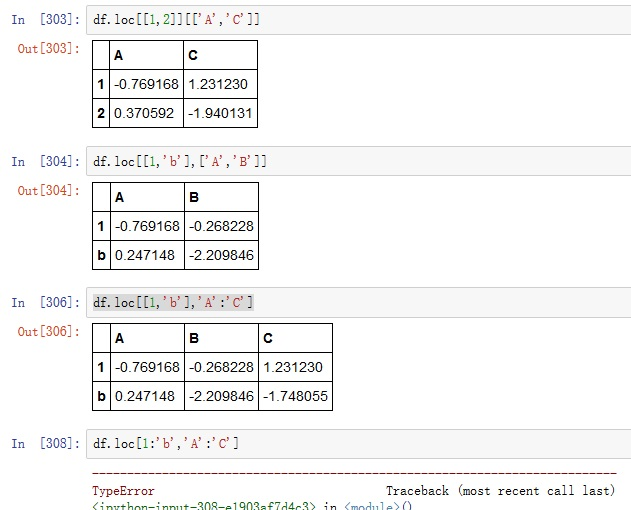ps：这里行号同样不能用切片，说明loc不支持行号切片索引。方法3：.iloc
(1)读取一个单元格
df.iloc[rowNo].col
df.iloc[rowNo][col]
df.iloc[rowNo,colNo]ps：df.iloc[rowNo,col]不支持。
(2)读取一行多列
df.iloc[rowNo,firestColNo,endColNo]
df.iloc[rowNo][[col1,col2]]
df.iloc[rowNo][firesCol:endCol]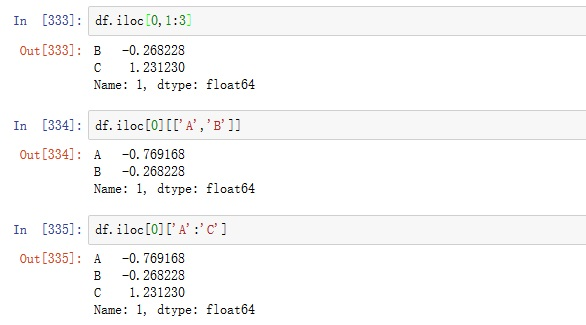ps：df.iloc[rowNo,[col1,col2]],df.iloc[rowNo,firstColNo:endColNo]均不支持。
(3)读取多行一列
df.iloc[[rowNo1,rowNo2],colNo]
df.iloc[firstRowNo:endRowNo,colNo]
df.iloc[[rowNo1,rowNo2]][col]
df.iloc[firstRowNo,endRowNo][col]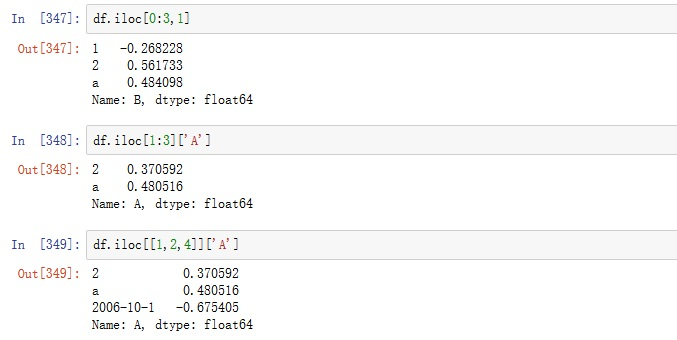(4)读取多行多列
df.iloc[firstRowNo:endRowNo,firstColNo:endColNo]
df.iloc[[RowNo1,RowNo2],[ColNo1,ColNo2]]
df.iloc[firstRowNo:endRowNo][[col1,col2]]方法4：.ix
累的不行了，这里就直接写多行多列的读取吧。
df.ix[firstRow/firstRowNo:endRow/endRowNo,firstCol/firstColNo:endCol/endColNo]
df.ix[[row1/rowNo1,row2,rowNo2],[col1/colNo1,col2,colNo2]]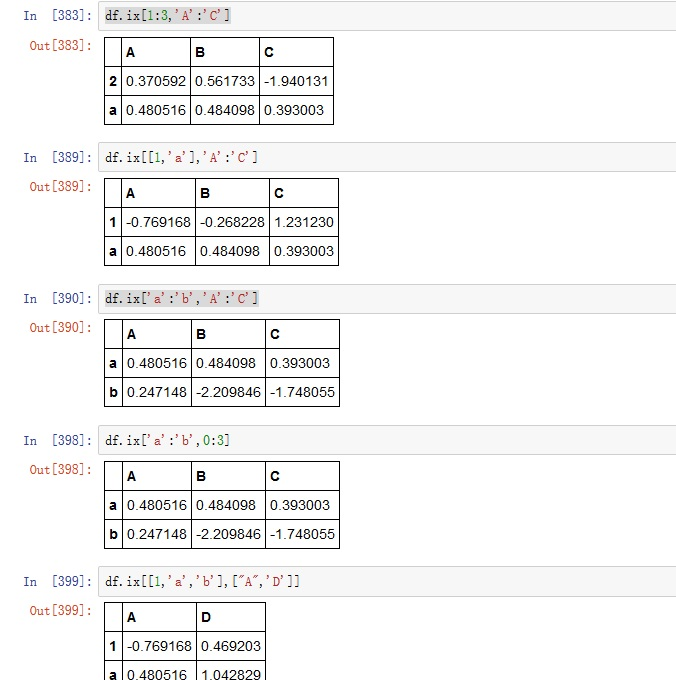方法5：	at,iat
3 写DataFrame/DataFrame赋值
3.1 按列赋值
df.col=colList/colValue
df[col]=colList/colValue
eg. df.A=[1,2,3,4,5,6],df['A']=0ps1：如果用一个列表或数组赋值，其长度必须和df的行数相同。
3.2 按行赋值
df.loc[row]=rowList
df.loc[row]=rowValue
3.3 给多行多列赋值
df.loc[[row1,row2],[col1,col2]]=value/valueList
df.iloc[[rowNo1,rowNo2],[colNo1,colNo2]]=value/valueList
df.iloc[[rowNo1,rowNo2]][[col1,col2]]=value/valueList
df.ix[firstRow:endRow,firstCol:endCol]=value/valueList
ps：DataFrame的读写操作是多变的，这里也仅仅列出了几种常用的方法，熟练一种方式即可。
4 DataFrame的插入
以下面DataFrame为例展开说明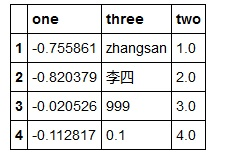4.1在任意位置插入
插入一列
insert(ioc,column,value)
ioc:要插入的位置
colunm:列名
value:值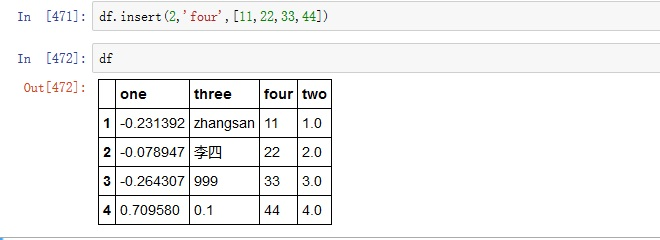插入一行
row={'one':111,'two':222,'three':333}
df.loc=row or
df.iloc=row or
df.ix=row or

4.2在末尾插入
如果插入一行或一列，用上面的方法把插入位置改为末尾即可，下面给出插入多行多列的方法。
pandas.concat(objs, axis=0, join_axes=None, ignore_index=False)
objs:合并对象
axis:合并方式，默认0表示按列合并，1表示按行合并
ignore_index:是否忽略索引

有df1和df2定义如下：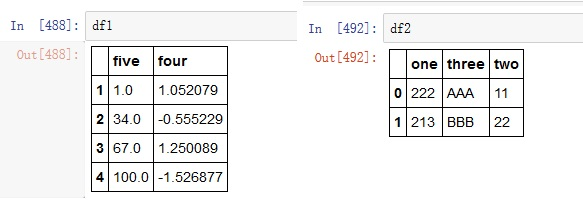按行合并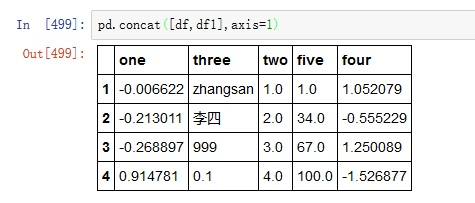按列合并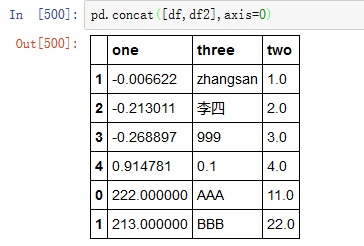利用append函数可以完成相同的操作: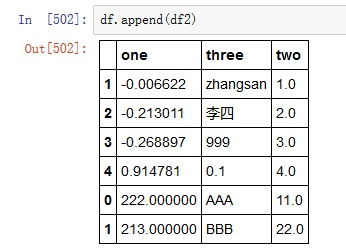5 DataFrame的删除操作
drop(labels, axis=0, level=None, inplace=False)
lables：要删除数据的标签
axis：0表示删除行，1表示删除列，默认0
inplace:是否在当前df中执行此操作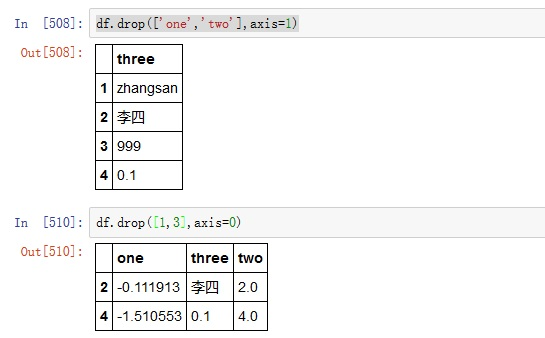后记：关于pandas的基本操作就写到这里了，关于以上内容如果有什么问题可以通过邮件联系博主，水平有限，请大家批评指正。
后面如果有机会，我会写关于DataFrame更进一步的操作，我也不知道会不会写，能写多少，先附上地址pandas进阶：DataFrame高级操作


展开全文pandas python
• 用法：DataFrame.drop(labels=None,axis=0, index=None, columns=None, inplace=False) 在这里默认：axis=0，指删除index，因此删除columns时要指定axis=1； inplace=False，默认该删除操作不改变原数据，而是返回...
用法：DataFrame.drop(labels=None,axis=0, index=None, columns=None, inplace=False)
参数说明：
labels 就是要删除的行列的名字，用列表给定
axis 默认为0，指删除行，因此删除columns时要指定axis=1；
index 直接指定要删除的行
columns 直接指定要删除的列
inplace=False，默认该删除操作不改变原数据，而是返回一个执行删除操作后的新dataframe；
inplace=True，则会直接在原数据上进行删除操作，删除后无法返回。
因此，删除行列有两种方式：
1）labels=None,axis=0  的组合
2）index或columns直接指定要删除的行或列
例子：
>>>df = pd.DataFrame(np.arange(12).reshape(3,4), columns=['A', 'B', 'C', 'D'])

>>>df

A   B   C   D

0  0   1   2   3

1  4   5   6   7

2  8   9  10  11

#Drop columns,两种方法等价

>>>df.drop(['B', 'C'], axis=1)

A   D

0  0   3

1  4   7

2  8  11

>>>df.drop(columns=['B', 'C'])

A   D

0  0   3

1  4   7

2  8  11

# 第一种方法下删除column一定要指定axis=1,否则会报错
>>> df.drop(['B', 'C'])

ValueError: labels ['B' 'C'] not contained in axis

#Drop rows
>>>df.drop([0, 1])

A  B   C   D

2  8  9  10  11

>>> df.drop(index=[0, 1])

A  B   C   D

2  8  9  10  11

Life is short, You need Python~


展开全文pandas drop
• ## pandas DataFrame数据转为list

万次阅读 多人点赞 2017-05-21 22:46:10
首先使用np.array()函数把DataFrame转化为np.ndarray()，再利用tolist()函数把np.ndarray()转为list，示例代码如下：# -*- coding:utf-8-*- import numpy as np import pandas as pddata_x = pd.read_csv("E:/...csv numpy 数据
• ## Python在Dataframe中新添加一列

万次阅读 多人点赞 2019-08-13 16:31:25
在敲代码的过程中，老是会遇到在Dataframe中新添加一列的情况，每次都要重新google，这次做个记录。 其实在Dataframe中新添加一列很简单，直接指明列名，然后赋值就可以了。 import pandas as pd data = pd....python
• ## Pandas：DataFrame对象的基础操作

万次阅读 多人点赞 2017-07-18 22:36:17
DataFrame对象的创建，修改，合并import pandas as pd import numpy as np创建DataFrame对象# 创建DataFrame对象 df = pd.DataFrame([1, 2, 3, 4, 5], columns=['cols'], index=['a','b','c','d','e']) df ....Pandas
• ## Pandas把dataframe或series转换成list

万次阅读 多人点赞 2019-08-12 12:25:15
dataframe转换为list 输入多维dataframe： df = pd.DataFrame({'a':[1,3,5,7,4,5,6,4,7,8,9], 'b':[3,5,6,2,4,6,7,8,7,8,9]}) 把a列的元素转换成list： # 方法1 df['a'].values.tolist() # 方法2 df['a']....Pandas series list
• ## pandas的dataframe如何更改数据类型？

万次阅读 多人点赞 2018-07-22 20:38:16
pandas的dataframe数据类型转换 在使用pandas库进行数据分析时，有时候会需要将object类型转换成数值类型(float,int)，那么如何做呢？ 主要有以下三种方法：创建时指定类型，df.astype强制类型转换，以及使用pd....
• ## python将字典转换成dataframe数据框

万次阅读 多人点赞 2018-12-17 18:13:54
需要将字典转换成dataframe来操作，并且将字典的key，value分别作为dataframe两列。 数据样例如下： 一个key只有一个value的字典如果直接转化成数据框会报错： 如下两种方法可达成目标。 一，将字典转换成...python 字典
• 1.删除/选取某列含有特殊数值的行 import pandas as pd import numpy as np ...df1=pd.DataFrame(a,index=['row0','row1','row2'],columns=list('ABC')) print(df1) df2=df1.copy() #删除/选取...python pandas
• ## pandas的DataFrame的append方法详细介绍

万次阅读 多人点赞 2018-12-11 22:18:06
官方文档介绍链接：append方法介绍 ...功能说明：向dataframe对象中添加新的行，如果添加的列名不在dataframe对象中，将会被当作新的列进行添加 other：DataFrame、series、dict、list这样的数据结构 i...
• 作者：lianghc ... ...pandas提供了一个类似于关系数据库的连接(join)操作的方法merage,可以根据一个或多个键将不同DataFrame中的行连接起来 语法如下： merge(left, right, how='inner', on=No...python pandas merge concat join
• ## Python中DataFrame按照行遍历

万次阅读 多人点赞 2017-09-07 13:49:21
在做分类模型时候，需要在DataFrame中按照行获取数据以便于进行训练和测试。import pandas as pddict=[[1,2,3,4,5,6],[2,3,4,5,6,7],[3,4,5,6,7,8],[4,5,6,7,8,9],[5,6,7,8,9,10]] data=pd.DataFrame(dict) print...
• 对于这个问题，相信很多人都会很困惑，本篇文章将会给大家介绍一种非常简单的方式向DataFrame中任意指定的位置添加一列。 在此之前或许有不少读者已经了解了最普通的添加一列的方式，如下： import pandas as pd...python
• iterrows(): 按行遍历，将DataFrame的每一行迭代为(index, Series)对，可以通过row[name]对元素进行访问。 itertuples(): 按行遍历，将DataFrame的每一行迭代为元祖，可以通过row[name]对元素进行访问，比iterrows()...
• DataFrame是Python中Pandas库中的一种数据结构，它类似excel，是一种二维表。 文章目录==DataFrame是Python中Pandas库中的一种数据结构，它类似excel，是一种二维表。==0 简介1 创建DataFrame1.1 使用numpy函数创建...pandas
• 这个repo 用来记录一些python技巧、书籍...用pandas中的DataFrame时选取行或列： import numpy as np import pandas as pd from pandas import Sereis, DataFrame ser = Series(np.arange(3.)) data = DataFrame...python pandas Series
• DataFrame是一种不可变的分布式数据集，这种数据集被组织成指定的列，类似于关系数据库中的表。SchemaRDD作为Apache Spark 1.0版本中的实验性工作，它在Apache Spark 1.3版本中被命名为DataFrame。对于熟悉Python ...
• @DataFrame操作 生成空dataframe m = pd.DataFrame() 生成一列全是0的array： m = np.zeros((4500,0)) 合并两个dataframe axis=1为列合并，列增加 axis=0为行合并，行增加 concat([df1,df2],axis=1)
• ## Pandas.DataFrame转置

万次阅读 多人点赞 2019-06-02 22:52:19
简述 Motivation sometimes，换一种获取数据的方式，可以提高数据获取的速度。...这些情况下，你可能就会需要遇到DataFrame行列转置的方法。 Contribution 提供了Pandas.DataFrame的行列转置的方法 实验部分...pandas
• Dataframe中的时间是不能直接进行相加减的。如果将两列时间进行加减，会弹出类型错误： TypeError: unsupported operand type(s) for -: 'str' and 'str' 所以需要先用pandas的to_datetime()方法，转化成时间...
• Spark DataFrame 与Pandas DataFrame差异为何使用pyspark dataframepandas dataframe数据结构特性spark dataframe结构与存储特性spark toPandas详解参考文献 为何使用pyspark dataframe 使用pandas进行数据处理...spark pandas
• ## DataFrame的copy的用法

万次阅读 多人点赞 2018-10-12 22:11:26
pandas.DataFrame.copy¶ DataFrame.copy(deep=True) 当deep=false相当于引用，原值改变复制的结果随着改变。 data=DataFrame.copy(deep=True) 等价于 data=DataFrame 假设有DataFrame： data.loc[["a"...
• 成功解决AttributeError: 'DataFrame' object has no attribute 'reshape' 目录 解决问题 解决思路 解决方法 解决问题 AttributeError: 'DataFrame' object has no attribute 'reshape' 解决思路...
• ## pandas dataframe 提取行和列

万次阅读 多人点赞 2019-01-11 11:27:09
data = pd.DataFrame({'a':[1,2,3],'b':[4,5,6],'c':[7,8,9]}) 提取列 单列 data['a'] 多列 data[['a', 'b']] 使用 .loc或者 .iloc 提取 第一个参数是行，第二个参数为列 .loc为按标签提取， .iloc为...python pandas
• Pandas DataFrame 对某一列求和 在操作pandas的DataFrame的时候，常常会遇到某些列是字符串，某一些列是数值的情况，如果直接使用df_obj.apply(sum)往往会出错 使用如下方式即可对其中某一列进行求和 dataf_test1['...
• ## 在pandas中遍历DataFrame行

万次阅读 多人点赞 2018-02-22 21:54:05
有如下 Pandas DataFrame：import pandas as pd inp = [{'c1':10, 'c2':100}, {'c1':11,'c2':110}, {'c1':12,'c2':120}] df = pd.DataFrame(inp) print df 上面代码输出： c1 c2 0 10 100 1 11 110 2 12 120 现在...
• ## Dataframe合并

千次阅读 2018-09-21 09:11:28
本文讲的是合并两个同列名的Dataframe数据，有两种方案。首先随意的产生两个通列名的Dataframe,如下所示： import pandas as pd from pandas import DataFrame df=DataFrame(np.arange(12).reshape((3,4)),index=...合并 pandas concat append
• ## 用pandas中的DataFrame时选取行或列

万次阅读 多人点赞 2017-12-24 21:59:58
import numpy as np import pandas as pd ...from pandas import Sereis, DataFrame ser = Series(np.arange(3.)) data = DataFrame(np.arange(16).reshape(4,4),index=list('abcd'),columns=list...